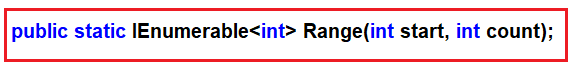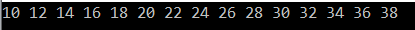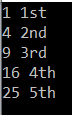# Linq Range Method in C#

## Linq Range Method in C# with Examples

In this article, I am going to discuss the Linq Range Method in C# with some examples. The Linq Range Method belongs to the Generation Operator category. So let us first discuss what Generation Operator is and then we will see the Range method. Please read our previous article before proceeding to this article where we discussed How to Implement Paging using LINQ Skip and Take method.

#### What is Generation Operator?

As of now, we have discussed so many LINQ extension methods such as Select, Where, Any, etc. The Enumerable class also provides three non-extension methods are as follows.

1. Range()
2. Repeat<T>()
3. Empty<T>()

These methods allow us to create some specific type of array, sequence, or collection using a single expression instead of creating them manually and populating them using some kind of loops. That means these methods return a new sequence or collection that implements the IEnumerable<T> interface.

Note: In this article, I am going to discuss the LINQ Range Method and the rest two methods are going to discuss in our upcoming articles.

##### Linq Range Method in C#:

This method is used to Generates a sequence of integral (integer) numbers within a specified range. The following is the signature of this method.This method has 2 integer parameters. The first parameter (i.e. int start) specifies the value of the first integer in the sequence. The second parameter (i.e. int count) specifies the number of sequential integers to be generated. The return type of this method is IEnumerable<int> which going to contain a range of sequential integer numbers.

Note: When the count value is less than 0 or when the start + count – 1 value larger than the MaxValue then it will through ArgumentOutOfRangeException.

##### Example1:

The following example generates a sequence of integers from 1 to 10 using the Linq Range method.

```using System.Linq;
using System;
using System.Collections.Generic;

namespace LinqDemo
{
class Program
{
static void Main(string[] args)
{
IEnumerable<int> numberSequence = Enumerable.Range(1, 10);

foreach (int i in numberSequence)
{
Console.WriteLine(i);
}

}
}
}```

Once you run the application, it will print the values from 1 to 10 as expected.

##### Example2: Range method with Filter.

The following print all the even numbers which are present between 10 and 30. Here, first we need to generate the sequence using range method and then we need to filter the data using the Where extension method.

```using System.Linq;
using System;
using System.Collections.Generic;

namespace LinqDemo
{
class Program
{
static void Main(string[] args)
{
IEnumerable<int> EvenNumbers = Enumerable.Range(10, 30).Where(x => x%2 == 0);

foreach (int num in EvenNumbers)
{
Console.Write(\$"{num} ");
}

}
}
}
```

Output:

#####Example3:

The following example shows how to generate a sequence of integers from 1 to 5 and then return the square of each number.

```using System.Linq;
using System;
using System.Collections.Generic;

namespace LinqDemo
{
class Program
{
static void Main(string[] args)
{
IEnumerable<int> EvenNumbers = Enumerable.Range(1, 5).Select(x => x*x);

foreach (int num in EvenNumbers)
{
Console.Write(\$"{num} ");
}

}
}
}
```

Output: 1 4 9 16 25

##### Example4: Range with string

In the following example first, we generate a sequence and then we pass the sequence to our CustomLogic method and then merge the sequence with the return value from the CustomLogic method and return the result as IEnumerable<string>.

```using System.Linq;
using System;
using System.Collections.Generic;

namespace LinqDemo
{
class Program
{
static void Main(string[] args)
{
IEnumerable<string> rangewithString = Enumerable.Range(1, 5).Select(x => (x * x) + " " + CustomLogic(x)).ToArray();

foreach (var item in rangewithString)
{
Console.WriteLine(item);
}

}

private static string CustomLogic(int x)
{
string result = string.Empty;
switch (x)
{
case 1:
result = "1st";
break;
case 2:
result = "2nd";
break;
case 3:
result = "3rd";
break;
case 4:
result = "4th";
break;
case 5:
result = "5th";
break;
}
return result;
}
}
}
```

Output:Note: This method is implemented by using the deferred execution. The immediate return value is an object that stores all the information that is required to perform the action. The query represented by this method is not executed until the object is enumerated either by calling its GetEnumerator method directly or by using a for each loop.

In the next article, I am going to discuss the Linq Repeat Method with some examples. Here, in this article, I try to explain the LINQ Range Method in C# with some different types of examples. I hope you understood the need and use of the Range method in Linq.